# 读论文——Structural Similarity Search for Formulas Using Leaf-Root Paths in Operator Subtrees

1. 如何把公式转化为操作树？
2. 与之前接触过的树相似度算法有何不同？

## 关键问题

• 以何种形式表达数学公式？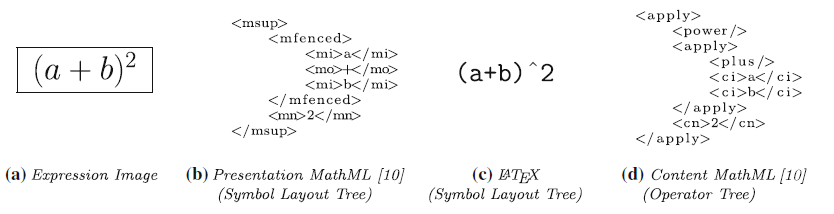• 数学公式的相似性如何衡量？

• 结构的相似性
• common subexpression：是否都存在相同的子表达式
• operator commutativity：交换律
• operator associativity：结合律
• 符号的相似性
• $(1+1/n)^n$与$(1+1/x)^x$ 虽然符号不同，但是$n$和$x$都表示未知数，语义相同，所以这两个是相同的公式；
• $e=mc^2$与$y=ax^2$ 在考虑物理意义的条件下，是两个不同的公式；
• 语义的相似性
• 等效的公式$1/x$和$x^{-1}$，就和苹果与apple一样

## 方法

• 比较树的结构
• 比较节点的名称

### 定义1 公共子树

$$CFS\left(T_{q}, T_{d}\right)=\left\{\hat{T}_{q}, \hat{T}_{d}: \hat{T}_{q} \preceq_{l} T_{q}, \hat{T}_{d} \preceq_{l} T_{d}, \hat{T}_{q} \cong \hat{T}_{d}, \hat{T}_{q} \subseteq T_{q}, \hat{T}_{d} \subseteq T_{d}\right\}$$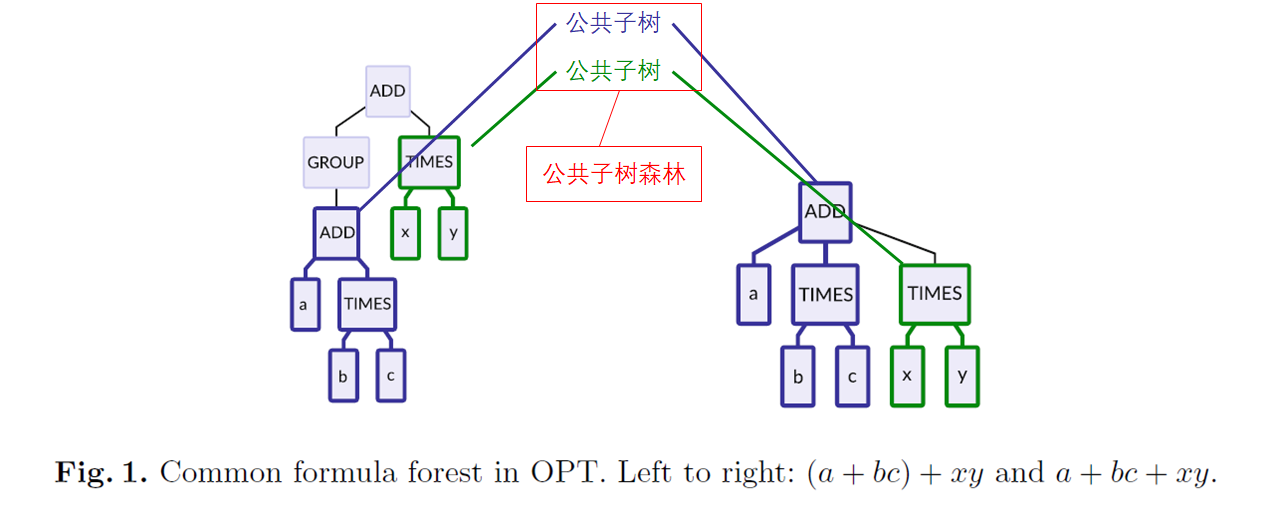### 定义2 公共子树森林

$$\pi=\left\{\left(\hat{T}_{q}^{1}, \hat{T}_{d}^{1}\right),\left(\hat{T}_{q}^{2}, \hat{T}_{d}^{2}\right), \ldots\left(\hat{T}_{q}^{n}, \hat{T}_{d}^{n}\right)\right\} \in \Pi\left(T_{q}, T_{d}\right)\qquad(1)$$

### 定义3 度量相似度

$$\Gamma_{\gamma}\left(T_{q}, T_{d}\right)=\max _{\pi \in \Pi\left(T_{q}, T_{d}\right)} \gamma(\pi)\qquad(2)$$ $$\gamma(\pi)=\sum_{\left(\hat{T}_{q}^{i}, \hat{T}_{d}^{i}\right) \in \pi} \beta_{i} \cdot\left(\alpha \cdot \text { internals }\left(\hat{T}_{d}^{i}\right)+(1-\alpha) \cdot \operatorname{leaves}\left(\hat{T}_{d}^{i}\right)\right)\qquad(3)$$
• $internals \left(T\right)$： T中节点或操作符的数目

• $leaves(T)$：T中叶节点/运算对象的数目

• $\alpha$：大于0小于1，操作符贡献的权重

• $\beta_i$：大于0，子表达式贡献的权重，子表达式的范围越广，贡献的权重越大。实际计算中，对于一些范围小的子树，该值取0

### 子表达式匹配

#### 贪心算法

$$\gamma(\pi)=\sum_{\left(\hat{T}_{q}^{i}, \hat{T}_{d}^{i}\right) \in \pi} \beta_{i} \cdot{leaves}\left(\hat{T}_{d}^{i}\right)$$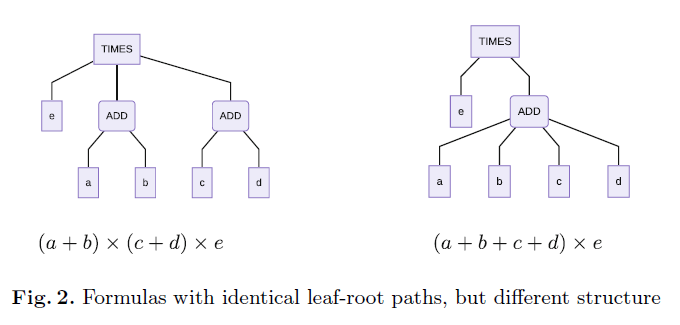1. 这种情况相对少见；
2. 希望设计一个更具有实用意义的搜索算法（快速）。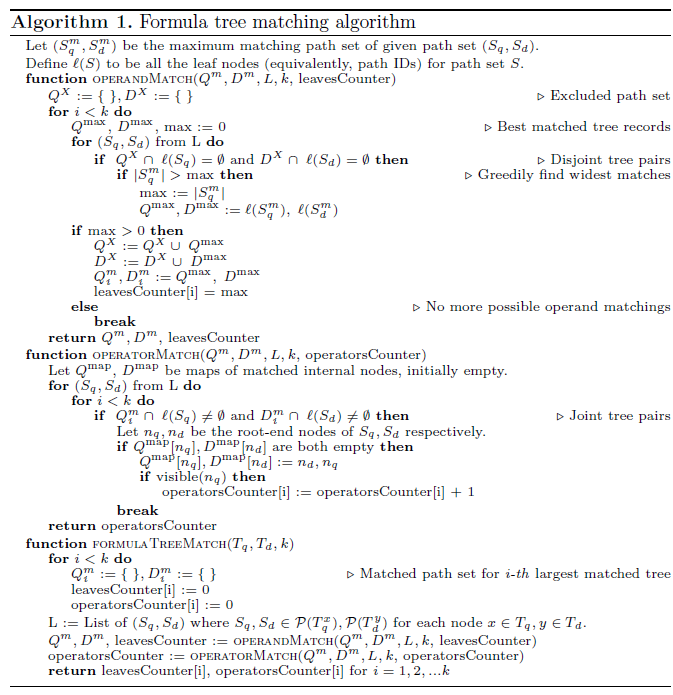### 检索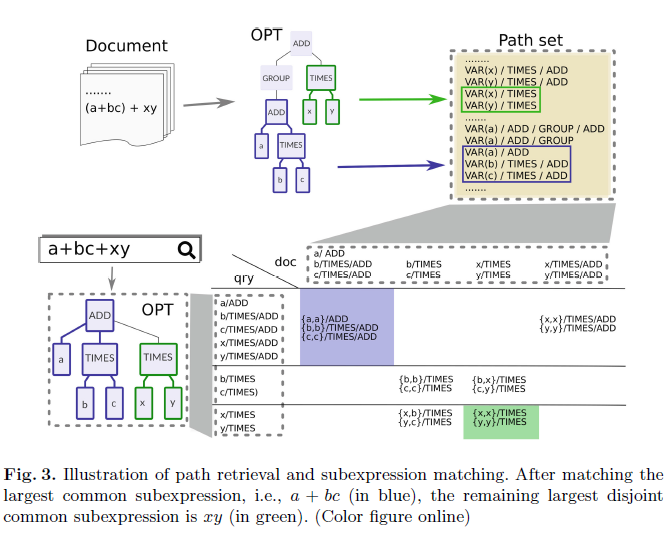1. 转化为操作树
2. 操作符，操作对象的令牌化：制定100+语法规则，50+符号类型。
3. 路径的生成：对于每一个操作符，都将其标记为根节点，记录所有叶节点到该节点的路径。
4. 记录的数据：路径的ID，操作符号和对象的ID

$$\frac{S_{ st } S_{ sy }}{S_{ st }+S_{ sy }}\left[(1-\theta)+\theta \frac{1}{\log \left(1+\operatorname{leaves}\left(T_{d}\right)\right)}\right], \quad \theta \in[0,1]\qquad(4)$$

$$S_{ st }= \begin{cases}\frac{\Gamma_{\gamma}\left(T_{q}, T_{d}\right)}{\operatorname{leaves}\left(T_{q}\right)} & \text { if } \alpha=0 \ \frac{\Gamma_{\gamma}\left(T_{q}, T_{d}\right)}{\operatorname{leaves}\left(T_{q}\right)+\text { internals }\left(T_{q}\right)} & \text { if } \alpha \neq 0\end{cases}\qquad(5)$$
$S_{sy}$是标准化的操作符号集的相似度，计算方式：
$$S_{ sy }=\frac{1}{1+(1-y)^{2}}\qquad(6)$$

in LaTeX markup and its implementation. Master’s thesis, University of Delaware）

## 总结与思考

• 这篇文章解决了哪些问题？

采用匹配叶根路径的方法，度量公式结构的相似度；使用贪心算法提高搜索的效率。

• 如何把公式转化为操作树？

这篇文章并没有详细描述转化为操作树的过程。查阅了这篇文章所引用的文献，对于将$\LaTeX$转化为树结构的问题，《Recognition and retrieval of mathematical expressions》这篇文章提到了，转化主要还是依靠制订一系列的语法规则。

• 与之前的树相似度算法有何不同？

考虑时间复杂度和搜索的效率。

目录